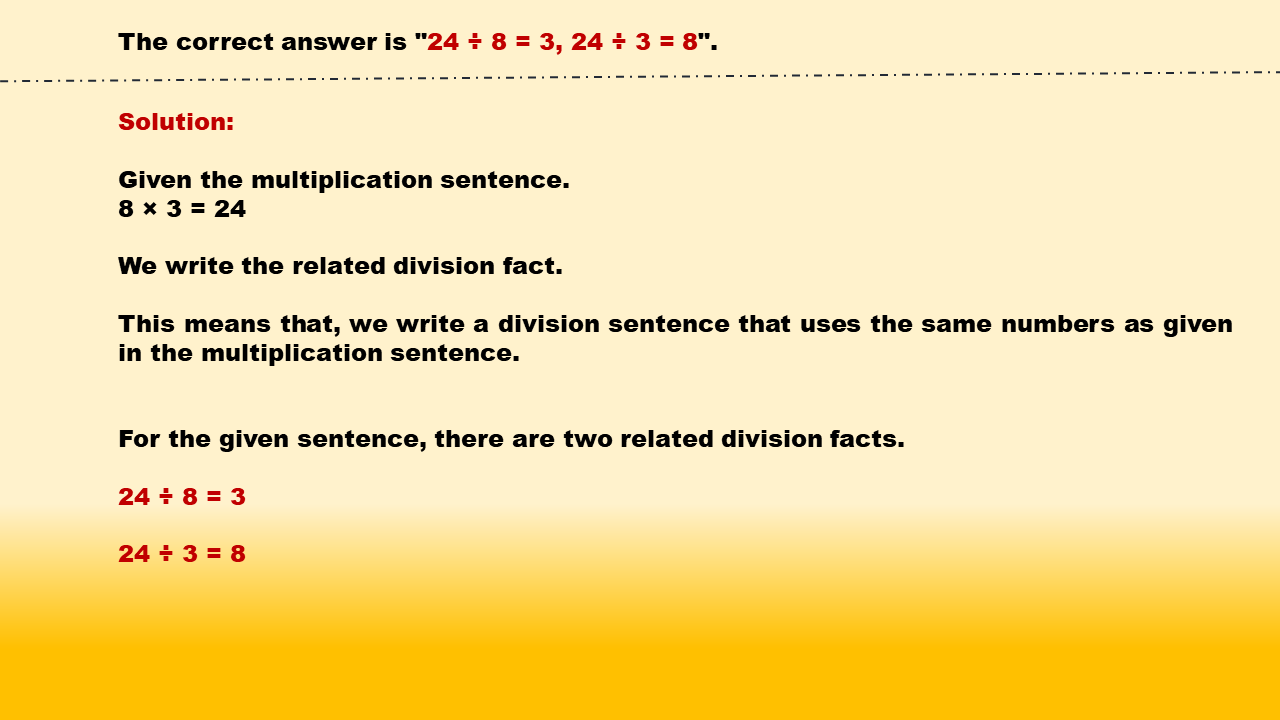1
visibility

Identify related division facts for 8 × 3 = 24.

• 60 ÷ 6 = 10, 60 ÷ 10 = 6

• 30 ÷ 6 = 5, 30 ÷ 5 = 6

• 24 ÷ 8 = 3, 24 ÷ 3 = 8

• 48 ÷ 8 = 6, 48 ÷ 6 = 8